## A UNIFIED COMPUTATIONAL FRAMEWORK FOR FLUID-SOLID COUPLING IN MARINE EARTHQUAKE ENGINEERING 1)

Chen Shaolin2), Ke Xiaofei, Zhang Hongxiang

Department of Civil Engineering, Nanjing University of Aeronautics and Astronautics, Nanjing 210016, China

 基金资助: 国家自然科学基金资助项目.  51178222国家自然科学基金资助项目.  51278260

Received: 2018-10-12   Accepted: 2018-12-18   Online: 2019-03-18

2)陈少林,教授,主要研究方向：地震工程.E-mail:icmcsl@nuaa.edu.cnAbstract

The simulation of seismic wavefield at seafloor and seismic response of marine structures involves the coupling between seawater, saturated seabed, elastic bedrock and structure. That means, we target simulation where several types of equations are involved such as fluid, solid and saturated porous media equation. The conventional method for this fluid-solid-saturated porous media interaction problem is to use exsisting solvers of different equations and coupling method, which needs data mapping, communication and coupling algorithm between different solvers. Here, we present an alternative method, in which the coulping between different solvers is avoided. In fact, when porosity equals to one and zero, the saturated porous media is reduced to fluid and solid respectively, so we can use the porous media equation to describe the ideal fluid and solid, and the coupling between porous media, solid and fluid turns to the coupling between porous media with different porosity. Based on this idea, firstly the Biot's equations are approximated by Galerkin scheme and the explicit lumped-mass FEM is chosen, that are well suited to parallel computation. Then considering the traction and velocity continuity on the interface between porous media with different porosity, the coupled algorithm is derived, which is proved to be suitable for the coupling between fluid,solid and saturated porous media. Thus, the coupling problem between fluid, solid and saturated porous media can be brought into a unified framework, in which only the solver of saturated porous media is used. The three-dimensional parallel code for this proposed method is programed, examples for analysis of layered water-saturated seabed, water-bedrock, and water-saturated seabed-bedrock semi-infinite systems subjected to plane P-SV wave are given, and the proposed unified framework is verified through comparison between the results obtained through the proposed unified framework combined with tansmitting boundary condition and those obtained through tansfer matrix method.

Keywords： fluid-solid coupling ; saturated porous medium ; marine earthquake engineering ; explicit lumped-mass finite element method ; transmitting boundary ; parallel computation

Chen Shaolin, Ke Xiaofei, Zhang Hongxiang. A UNIFIED COMPUTATIONAL FRAMEWORK FOR FLUID-SOLID COUPLING IN MARINE EARTHQUAKE ENGINEERING 1). Chinese Journal of Theoretical and Applied Mechanics[J], 2019, 51(2): 594-606 DOI:10.6052/0459-1879-18-333

## 引 言

### 图1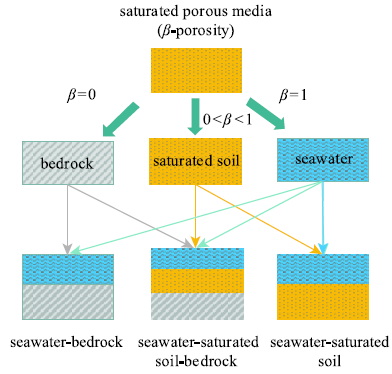Fig.1   Schematic diagram of media relations

## 1 基本理论

### 1.1 一般饱和多孔介质情形

${\bf{L}}_{\rm s}^{\rm T} {{{\sigma }'}}-(1-\beta ){\bf{L}}_{\rm w}^{\rm T} P + b({{\dot {U}}}-{{\dot {u}}}) = (1-\beta )\rho _{\rm s} {{\ddot {u}}}$

$-\beta {\bf{L}}_{\rm w}^{\rm T} P + b({{\dot {u}}}-{{\dot {U}}}) = \beta \rho _{\rm w} {{\ddot {U}}}$

$$\tau =-\beta P = E_{\rm w} [\beta e^{\rm w} + (1-\beta )e^{\rm s}]$$

$${\bf{L}}_{\rm s} = \left[ {{\begin{array}{*{20}c} {\partial/ {\partial x_1 }} & 0 & 0 \\ 0 & {\partial/ {\partial x_2 }} & 0 \\ 0 & 0 & {\partial/ {\partial x_3}} \\ {\partial/{\partial x_2 }} & {\partial/ {\partial x_1 }} & 0 \\ 0 & {\partial /{\partial x_3 }} & {\partial /{\partial x_2 }} \\ {\partial/ {\partial x_3 }} & 0 & {\partial/ {\partial x_1 }} \\ \end{array} }} \right]$$

$${\bf{L}}_{\rm w} = \left( {\partial/ {\partial x_1 ,\partial / {\partial x_2 ,\partial/{\partial x_3 }}}} \right)$$

Dirichlet边界条件

$${u}-\bar{u} ={0}$$

$${{U}}-{{\bar {U}}} ={{{{0}}}}$$

Neumann边界条件

$$\hat {n}{{\sigma}}-{{\bar {\sigma }}} = {0}$$

$$(P-\bar {P}){{n}} ={\bf 0}$$

$${{n}} = \left( {n_x ,n_y ,n_z } \right)^{\rm T}$$

$${{\hat {n}}} = \left[ {{\begin{array}{*{20}c} {n_x } & 0 & 0 & {n_y } & 0 & {n_z } \\ 0 & {n_y } & 0 & {n_x } & {n_z } & 0 \\ 0 & 0 & {n_z } & 0 & {n_y } & {n_x } \\ \end{array} }} \right]$$

${{\ddot {u}}}_i {{M}}_{{\rm s}i} + {{F}}_i^{\rm s} + {{T}}_i^{\rm s}-{{S}}_i^{\rm s} = {\bf 0}$

${{\ddot {U}}}_i {{M}}_{{\rm w}i} + {{F}}_i^{\rm w} + {{T}}_i^{\rm w}-{{S}}_i^{\rm w} = {\bf 0}$

$${{M}}_{{\rm s}i} = \sum\limits_e {\sum\limits_{j = 1}^J {\int_{\mbox{ }\Omega ^e} {{{N}}_i^{\rm T} } (1-\beta )\rho _{\rm s} {{N}}_j {\rm d}V} }$$

$${{M}}_{{\rm w}i} = \sum\limits_e {\sum\limits_{j = 1}^J {\int_{\mbox{ }\Omega ^e} {{{N}}_i^{\rm T} } \beta \rho _{\rm w} {{ N}}_j {\rm d}V} }$$

$${{T}}_i^{\rm s} = \sum\limits_e {\int_{\mbox{ }var\Omega ^e} {{{N}}_i ^{\rm T}b{{N}}_j ({{\dot {u}}}_j-{{\dot {U}}}_j )} {\rm d}V}$$

$${{T}}_i^{\rm s} = \sum\limits_e {\int_{\mbox{ }\Omega ^e} {{{N}}_i ^{\rm T}b{{N}}_j ({{\dot {u}}}_j-{{\dot {U}}}_j )} {\rm d}V}$$

$${{T}}_i^{\rm s} = \sum\limits_e {\int_{{ }\Omega ^e} {{{N}}_i ^{\rm T}b{{N}}_j ({{\dot {u}}}_j-{{\dot {U}}}_j )} {\rm d}V}$$

$${{S}}_i^{\rm s} = \sum\limits_e {\int_{{S}^e} {{{N}}_i ^{\rm T}} {{\hat {n}\sigma }}{\rm d}S}$$

$${{S}}_i^{\rm s} = \sum\limits_e {\int_{{S}^e} {{{N}}_i ^{\rm T}} {{\hat {n}\sigma }}{\rm d}S}$$

$${{S}}_i^{\rm w} = \sum\limits_e {\int_{{S}^e} {{{N}}_i ^{\rm T}} {{n}}\beta P{\rm d}S}$$

### 图2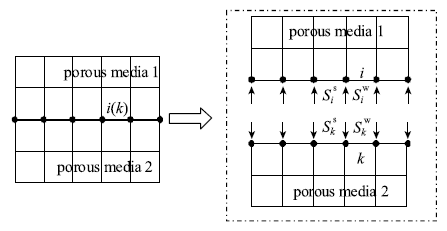Fig.2   Schematic diagram of interfacial force

${{\bar { \ddot {u}}}}_k {{\bar {M}}}_{{\rm s}k} + {{\bar {F}}}_k^{\rm s} + {{\bar {T}}}_k^{\rm s}-{{\bar {S}}}_k^{\rm s} = 0$

${{ \bar{\ddot {U}}}}_k {{\bar {M}}}_{{\rm w}k} + {{\bar { F}}}_k^{\rm w} + {{\bar {T}}}_k^{\rm w}-{{\bar {S}}}_k^{\rm w} = 0$

$${{\bar {M}}}_{{\rm s}k} = \sum\limits_e {\sum\limits_{j = 1}^J {\int_{\mbox{ }\Omega ^e} {{{N}}_k^{\rm T} } (1-\bar {\beta })\bar {\rho }_{\rm s} {{N}}_j{\rm d}V} }$$

$${{\bar {M}}}_{{\rm w}k} = \sum\limits_e {\sum\limits_{j = 1}^J {\int_{\mbox{ }\Omega ^e} {{{N}}_k^{\rm T} } \bar {\beta }\bar {\rho }_{\rm w} {{N}}_j {\rm d}V} }$$

$${{\bar {F}}}_k^{\rm s} = \sum\limits_e {{{\bar {f}}}_k^{{\rm s}e} } = \sum\limits_e {\int_{\mbox{ }\Omega ^e} {({\bf {L}}_{\rm s} {{N}}_k )^{\rm T}{{\bar {\sigma }}}} {\rm d}V}$$

$${{\bar {F}}}_k^{\rm w} = \sum\limits_e {{{\bar {f}}}_k^{we} } = \sum\limits_e {\int_{\mbox{ }\Omega ^e} {({\bf {L}}_{\rm w} {{N}}_k )^{\rm T}\bar {\beta }\bar {P}} {\rm d}V}$$

$${{\bar {T}}}_k^{\rm s} = \sum\limits_e {\int_{\mbox{ }\Omega ^e} {{{N}}_k ^{\rm T}\bar {b}{{N}}_j ({{\dot {u}}}_j-{{\dot {U}}}_j )} {\rm d}V}$$

$${{\bar {T}}}_k^{\rm w} =-{{\bar {T}}}_k^{\rm s}$$

$${{\bar {S}}}_k^{\rm s} = \sum\limits_e {\int_{{ S}^e} {{{N}}_k ^{\rm T}} {{ \bar{\hat{n}}\bar {\sigma }}}{\rm d}S}$$

$${{\bar {S}}}_k^{\rm w} = \sum\limits_e {\int_{{ S}^e} {{{N}}_k ^{\rm T}} {{\bar {n}}}\bar {\beta }\bar {P}{\rm d}S}$$

$$\sigma _{zz} + \tau = \bar {\sigma }_{zz} + \bar {\tau }$$

$${\sigma _{zx}} = {\bar \sigma _{zx}}, {\sigma _{zy}} = {\bar \sigma _{zy}}$$

$$P-\bar {P} = {k}'\beta (\dot {U}_z-\dot {u}_z )$$

$$\dot {u}_x =\bar { \dot {u}}_x , \dot {u}_y = \dot {\bar {u}}_y$$

$$\dot {u}_z = \bar {\dot{u}}_z,~ \beta (\dot {U}_z-\dot {u}_z) = \bar {\beta }( \bar{\dot {U}}_z-\bar {\dot {u}}_z )$$

$\bar {\ddot{u}}_{{\rm k}x} (m_{{\rm s}i} + \bar {m}_{{\rm s}k} ) + F_{ix}^{\rm s} + \bar {F}_{kx}^{\rm s} + T_{ix}^{\rm s} + \bar {T}_{kx}^{\rm s} = 0$

$\bar {\ddot{u}}_{ky} (m_{{\rm s}i} + \bar {m}_{{\rm s}k} ) + F_{iy}^{\rm s} + \bar {F}_{ky}^{\rm s} + T_{iy}^{\rm s} + \bar {T}_{ky}^{\rm s} = 0$

$m_{{\rm w}i} \ddot {U}_{iy} + F_{iy}^{\rm w} + T_{iy}^{\rm w} = 0$

$m_{{\rm w}i} \ddot {U}_{iy} + F_{iy}^{\rm w} + T_{iy}^{\rm w} = 0$

$$\bar {m}_{{\rm w}k} \bar{\ddot {U}}_{kx} + \bar {F}_{kx}^{\rm w} + \bar {T}_{kx}^{\rm w} = 0$$

$$\bar {m}_{{\rm w}k} \bar{\ddot {U}}_{ky} + \bar {F}_{ky}^{\rm w} + \bar {T}_{ky}^{\rm w} = 0$$

$$\bar {m}_{{\rm s}k} \bar{\ddot {u}}_{kz} + \bar {m}_{{\rm w}k} \bar {\ddot {U}}_{kz} + m_{{\rm s}i} \ddot {u}_{iz} + m_{{\rm w}i} \ddot {U}_{iz} + F_{iz}^{\rm s} + F_{iz}^{\rm w} + \\ \bar {F}_{kz}^{\rm s} + \bar {F}_{kz}^{\rm w} + T_{iz}^{\rm s} + T_{iz}^{\rm w} + \bar {T}_{kz}^{\rm s} + \bar {T}_{kz}^{\rm w} = 0$$

$$\bar {\beta }m_{{\rm w}i} \ddot {U}_{iz} + \beta \bar {m}_{{\rm w}k} \bar {\ddot{U}}_{kz} + \bar {\beta }F_{iz}^{\rm w} + \beta \bar {F}_{kz}^{\rm w} +\\ \bar {\beta }T_{iz}^{\rm w} + \beta \bar {T}_{kz}^{\rm w} = 0$$

$$A_{11} \bar{\ddot {u}}_{kz} + A_{12} \bar{\ddot{U}}_{kz} + B_{11} = 0$$

$$A_{21} \bar{\ddot {u}}_{kz} + A_{22} \bar{\ddot {U}}_{kz} + B_{22} = 0$$

$$A_{11} = m_{{\rm s}i} + \bar {m}_{{\rm s}k} + (1-\bar {\beta }/\beta )m_{{\rm w}i}$$

$$A_{12} = \bar {m}_{{\rm w}k} + ({\bar {\beta }} / \beta )m_{{\rm w}i}$$

$$A_{21} = \bar {\beta }(1-\bar {\beta }/ \beta )m_{{\rm w}i}$$

$$A_{22} = \beta \bar {m}_{{\rm w}k} + (\bar {\beta }^2 / \beta)m_{{\rm w}i} + \varepsilon$$

$$B_{11} = F_{iz}^{\rm s} + F_{iz}^{\rm w} + \bar {F}_{kz}^{\rm s} +\bar {F}_{kz}^{\rm w} + T_{iz}^{\rm s}+\\ T_{iz}^{\rm w} + \bar {T}_{kz}^{\rm s} + \bar {T}_{kz}^{\rm w}$$

$$B_{22} = \bar {\beta }F_{iz}^{\rm w} + \beta \bar {F}_{kz}^{\rm w} + \bar {\beta }T_{iz}^{\rm w} + \beta \bar {T}_{kz}^{\rm w}$$

$$\bar{\ddot {u}}_{kz} = \frac{A_{22} B_{11}-A_{12} B_{22} }{A_{21} A_{12}-A_{11} A_{22} }$$

$$\bar{\ddot {U}}_{kz} = \frac{A_{11} B_{22}-A_{21} B_{11} }{A_{21} A_{12}-A_{11} A_{22} }$$

$$\ddot {U}^p = \frac{1}{\Delta t^2}(U^{(p + 1)}-2U^p + U^{(p-1)})$$

$$\dot {U}^p = \frac{1}{\Delta t}(U^p-U^{(p-1)})$$

$$\bar {u}_{kx}^{(p + 1)} = 2\bar {u}_{kx}^p-\bar {u}_{kx}^{(p-1)}-\frac{\Delta t^2}{(m_{si} + \bar {m}_{sk} )}(F_{ix}^{{\rm s}p} +\\ \bar {F}_{kx}^{{\rm s}p} + T_{ix}^{{\rm s}p} + \bar {T}_{kx}^{{\rm s}p} )$$

$$\bar {u}_{ky}^{(p + 1)} = 2\bar {u}_{ky}^p-\bar {u}_{ky}^{(p-1)}-\frac{\Delta t^2}{(m_{si} + \bar {m}_{sk} )}(F_{iy}^{{\rm s}p} +\\ \bar {F}_{ky}^{{\rm s}p} + T_{iy}^{{\rm s}p} + \bar {T}_{ky}^{{\rm s}p} )$$

$$\bar {u}_{kz}^{(p + 1)} = 2\bar {u}_{kz}^p-\bar {u}_{kz}^{(p-1)} + \frac{A_{22} B_{11}^p-A_{12} B_{22}^p }{A_{21} A_{12}-A_{11} A_{22} }\Delta t^2$$

$$\bar {U}_{kx}^{(p + 1)} = 2\bar {U}_{kx}^p-\bar {U}_{kx}^{(p-1)}-\frac{\Delta t^2}{\bar {m}_{{\rm w}k} }(\bar {F}_{kx}^{{\rm w}p} + \bar {T}_{kx}^{{\rm w}p} )$$

$$\bar {U}_{ky}^{(p + 1)} = 2\bar {U}_{ky}^p-\bar {U}_{ky}^{(p-1)}-\frac{\Delta t^2}{\bar {m}_{{\rm w}k} }(\bar {F}_{ky}^{{\rm w}p} + \bar {T}_{ky}^{{\rm w}p} )$$

$$\bar {U}_{kz}^{(p + 1)} = 2\bar {U}_{kz}^p-\bar {U}_{kz}^{(p-1)} + \frac{A_{11} B_{22}^p-A_{21} B_{11}^p }{A_{21} A_{12}-A_{11} A_{22} }\Delta t^2$$

$$U_{ix}^{(p + 1)} = 2U_{ix}^p-U_{ix}^{(p-1)}-\frac{\Delta t^2}{m_{{\rm w}i} }(F_{ix}^{{\rm w}p} + T_{ix}^{{\rm w}p} )$$

$$U_{iy}^{(p + 1)} = 2U_{iy}^p-U_{iy}^{(p-1)}-\frac{\Delta t^2}{m_{{\rm w}i} }(F_{iy}^{{\rm w}p} + T_{iy}^{{\rm w}p} )$$

$$u_{ix}^{(p + 1)} = \bar {u}_{kx}^{(p + 1)},~ u_{iy}^{(p + 1)} = \bar {u}_{ky}^{(p + 1)},~ u_{iz}^{(p + 1)} = \bar {u}_{kz}^{(p + 1)}$$

$$U_{iz}^{(p + 1)} = \bar {\beta }/ \beta (\bar {U}_{kz}^{(p + 1)}-\bar {u}_{kz}^{(p + 1)} ) + \bar {u}_{kz}^{(p + 1)}$$

### 1.2 特殊情形

1.2.1 流体-饱和土情形

${{\ddot {U}}}_i {{M}}_{{\rm w}i} + {{F}}_i^{\rm w}-{{S}}_i^{\rm w} = {\bf 0}$

$$P = \bar {\sigma }_{zz} + \bar {\tau }$$

$$P = \bar {P}$$

$$\dot {U}_z = \bar {\beta }(\bar{\dot {U}}_z-\bar{\dot {u}}_z ) + \dot {\bar {u}}_z$$

$$\bar {u}_{kx}^{(p + 1)} = 2\bar {u}_{kx}^p-\bar {u}_{kx}^{(p-1)}-\frac{\Delta t^2}{\bar {m}_{{\rm s}k} }(\bar {F}_{kx}^{{\rm s}p} + \bar {T}_{kx}^{{\rm s}p} )$$

$$\bar {u}_{ky}^{(p + 1)} = 2\bar {u}_{ky}^p-\bar {u}_{ky}^{(p-1)}-\frac{\Delta t^2}{\bar {m}_{{\rm s}k} }(\bar {F}_{ky}^{{\rm s}p} + \bar {T}_{ky}^{{\rm s}p} )$$

$$\bar {u}_{kz}^{(p + 1)} = 2\bar {u}_{kz}^p-\bar {u}_{kz}^{(p-1)} + \frac{A_{22} B_{11}^p-A_{12} B_{22}^p }{A_{21} A_{12}-A_{11} A_{22} }\Delta t^2$$

$$\bar {U}_{kx}^{(p + 1)} = 2\bar {U}_{kx}^p-\bar {U}_{kx}^{(p-1)}-\frac{\Delta t^2}{\bar {m}_{{\rm w}k} }(\bar {F}_{kx}^{{\rm w}p} + \bar {T}_{kx}^{{\rm w}p} )$$

$$\bar {U}_{ky}^{(p + 1)} = 2\bar {U}_{ky}^p-\bar {U}_{ky}^{(p-1)}-\frac{\Delta t^2}{\bar {m}_{{\rm w}k} }(\bar {F}_{ky}^{{\rm w}p} + \bar {T}_{ky}^{{\rm w}p} )$$

$$\bar {U}_{kz}^{(p + 1)} = 2\bar {U}_{kz}^p-\bar {U}_{kz}^{(p-1)} + \frac{A_{11} B_{22}^p-A_{21} B_{11}^p }{A_{21} A_{12}-A_{11} A_{22} }\Delta t^2$$

$$U_{ix}^{(p + 1)} = 2U_{ix}^p-U_{ix}^{(p-1)}-\frac{\Delta t^2}{m_{{\rm w}i} }F_{ix}^{{\rm w}p}$$

$$U_{iy}^{(p + 1)} = 2U_{iy}^p-U_{iy}^{(p-1)}-\frac{\Delta t^2}{m_{{\rm w}i} }F_{iy}^{{\rm w}p}$$

$$U_{iz}^{(p + 1)} = \bar {\beta }(\bar {U}_{kz}^{(p + 1)}-\bar {u}_{kz}^{(p + 1)} ) + \bar {u}_{kz}^{(p + 1)}$$

$$A_{11} = \bar {m}_{{\rm s}k} + (1-\bar {\beta })m_{{\rm w}i}$$

$$A_{12} = \bar {m}_{{\rm w}k} + \bar {\beta }m_{{\rm w}i}$$

$$A_{21} = \bar {\beta }(1-\bar {\beta })m_{{\rm w}i}$$

$$A_{22} = \bar {m}_{{\rm w}k} + \bar {\beta }^2m_{{\rm w}i}$$

$$B_{11}^p = F_{iz}^{{\rm w}p} + \bar {F}_{kz}^{{\rm s}p} + \bar {F}_{kz}^{{\rm w}p} + \bar {T}_{kz}^{{\rm s}p} + \bar {T}_{kz}^{{\rm w}p}$$

$$B_{22}^p = \bar {\beta }F_{iz}^{{\rm w}p} + \bar {F}_{kz}^{{\rm w}p} + \bar {T}_{kz}^{{\rm w}p}$$

1.2.2 饱和土-干基岩情形

${{\ddot { \bar {u}}}}_k {{\bar {M}}}_{{\rm s}k} + {{\bar {F}}}_k^{\rm s}-{{\bar {S}}}_k^{\rm s} = {\bf 0}$

$$\sigma _{zz} + \tau = \bar {\sigma }_{zz}$$

$${\sigma _{zx}} = {\bar \sigma _{zx}},~ {\sigma _{zy}} = {\bar \sigma _{zy}}$$

$$\dot {u}_x = \bar {\dot {u}}_x ,~ \dot {u}_y = \bar {\dot {u}}_y$$

$$\dot {u}_z = \bar {\dot {u}}_z ,~ \beta (\dot {U}_z-\dot {u}_z ) = 0$$

$$\bar {u}_{kx}^{(p + 1)} = 2\bar {u}_{kx}^p-\bar {u}_{kx}^{(p-1)}-{\Delta t^2}(F_{ix}^{{\rm s}p}+ \\ \bar {F}_{kx}^{{\rm s}p} + T_{ix}^{{\rm s}p} )/{(m_{{\rm s}i} + \bar {m}_{{\rm s}k} )}$$

$$\bar {u}_{ky}^{(p + 1)} = 2\bar {u}_{ky}^p-\bar {u}_{ky}^{(p-1)}-{\Delta t^2}(F_{iy}^{{\rm s}p}+\\ \bar {F}_{ky}^{{\rm s}p} + T_{iy}^{{\rm s}p} )/{(m_{{\rm s}i} + \bar {m}_{{\rm s}k} )}$$

$$\bar {u}_{kz}^{(p + 1)} = 2\bar {u}_{kz}^p-\bar {u}_{kz}^{(p-1)} + {B_{11}^p }\Delta t^2/{A_{11} }$$

$$U_{ix}^{(p + 1)} = 2U_{ix}^p-U_{ix}^{(p-1)}-{\Delta t^2}(F_{ix}^{{\rm w}p} + T_{ix}^{{\rm w}p} )/{m_{{\rm w}i} }$$

$$U_{iy}^{(p + 1)} = 2U_{iy}^p-U_{iy}^{(p-1)}-{\Delta t^2}(F_{iy}^{{\rm w}p} + T_{iy}^{{\rm w}p} )/{m_{ik} }$$

$$u_{ix}^{(p + 1)} = \bar {u}_{ix}^{(p + 1)}$$

$$u_{iy}^{(p + 1)} = \bar {u}_{iy}^{(p + 1)}$$

$$u_{iz}^{(p + 1)} = \bar {u}_{iz}^{(p + 1)}$$

$$U_{iz}^{(p + 1)} = \bar {u}_{iz}^{(p + 1)}$$

$$A_{11} = m_{{\rm s}i} + \bar {m}_{{\rm s}k} + m_{{\rm w}i}$$

$$B_{11}^p = F_{iz}^{{\rm s}p} + F_{iz}^{{\rm w}p} + \bar {F}_{kz}^{{\rm s}p} + T_{iz}^{{\rm s}p} + T_{iz}^{{\rm w}p}$$

1.2.3 流体-干基岩情形

$$P = \bar {\sigma }_{zz}$$

$$\dot {U}_z = \bar {\dot{u}}_z$$

$$\bar {u}_{kx}^{(p + 1)} = 2\bar {u}_{kx}^p-\bar {u}_{kx}^{(p-1)}-\frac{\Delta t^2}{\bar {m}_{{\rm s}k} }\bar {F}_{kx}^{{\rm s}p}$$

$$\bar {u}_{ky}^{(p + 1)} = 2\bar {u}_{ky}^p-\bar {u}_{ky}^{(p-1)}-\frac{\Delta t^2}{\bar {m}_{{\rm s}k} }\bar {F}_{ky}^{{\rm s}p}$$

$$\bar {u}_{kz}^{(p + 1)} = 2\bar {u}_{kz}^p-\bar {u}_{kz}^{(p-1)} + \frac{B_{11}^p }{A_{11} }\Delta t^2$$

$$U_{ix}^{(p + 1)} = 2U_{ix}^p-U_{ix}^{(p-1)}-\frac{\Delta t^2}{m_{{\rm w}i} }F_{ix}^{{\rm w}p}$$

$$U_{iy}^{(p + 1)} = 2U_{iy}^p-U_{iy}^{(p-1)}-\frac{\Delta t^2}{m_{{\rm w}i} }F_{iy}^{{\rm w}p}$$

$$U_{iz}^{(p + 1)} = \bar {u}_{iz}^{(p + 1)}$$

$$A_{11} = \bar {m}_{{\rm s}k} + m_{{\rm w}i}$$

$$B_{11}^p = F_{iz}^{{\rm w}p} + \bar {F}_{kz}^{{\rm s}p}$$

### 图3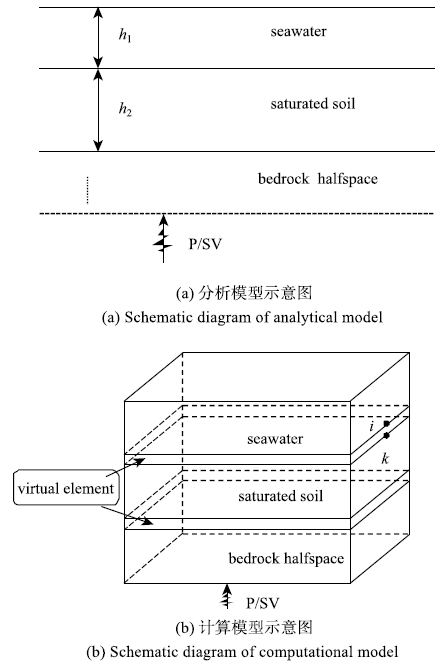Fig.3   Schematic diagram of model

## 2 算例验证

$${\sigma }'_{ij}-(1-\alpha )\delta _{ij} P = 2\mu e_{ij} + \delta _{ij} \lambda e$$

$$P =-\alpha Me + M\zeta$$

$$\alpha = 1-\frac{E_{\rm b} }{E_{\rm u} }$$

$$M = \frac{E_{\rm u}^2 }{E_{\rm u} \left[n\left(\frac{E_{\rm u} }{E_{\rm w} }-1\right) + 1\right]-E_{\rm b} }$$

### 图4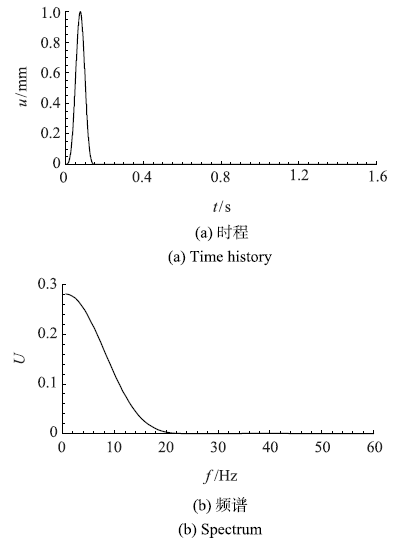Fig.4   Input pulse

Table 1  Parameters of material

MediaProsity ySPs/(kg.m_3)Pw/(kg.m_3)VG/MPaEw/GPaM/GPaak0 / ^m2
seawater10010000.4902.252.2511
saturated soil0.260.001200010000.4983.22.254.780.69710_7
bedrock00250000.2480000

### 图5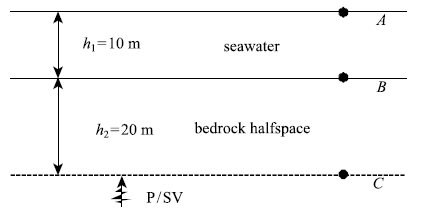Fig.5   Seawater-bedrock model

### 图6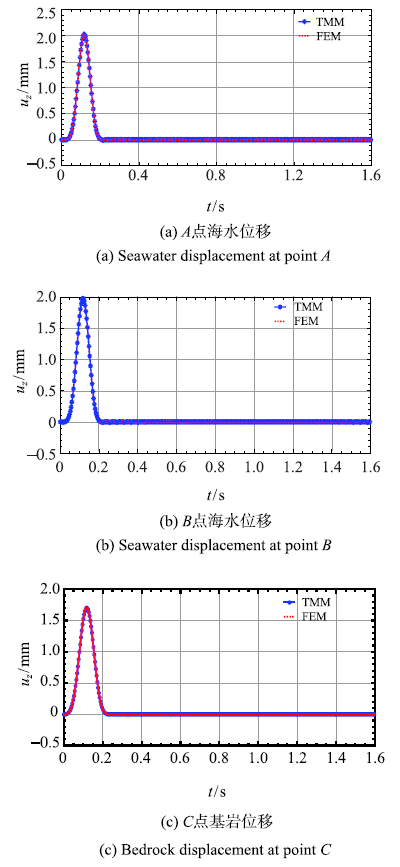Fig.6   Displacement of seawater-bedrock system for P wave incidence

### 图7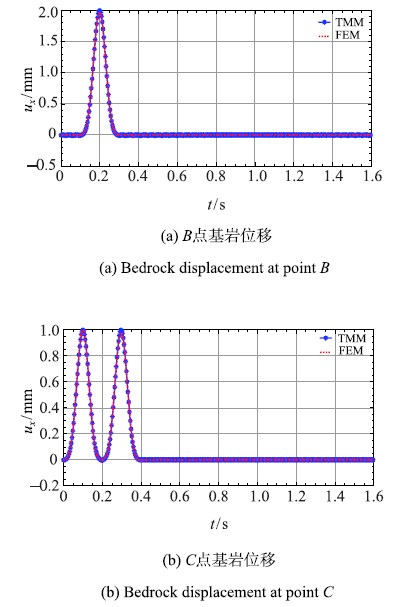Fig.7   Displacement of seawater-bedrock system for SV wave incidence

### 图8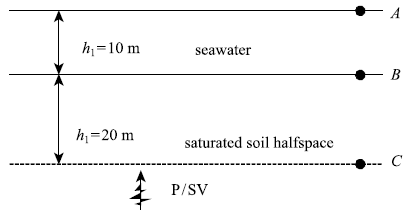Fig.8   Seawater-saturated soil model

### 图9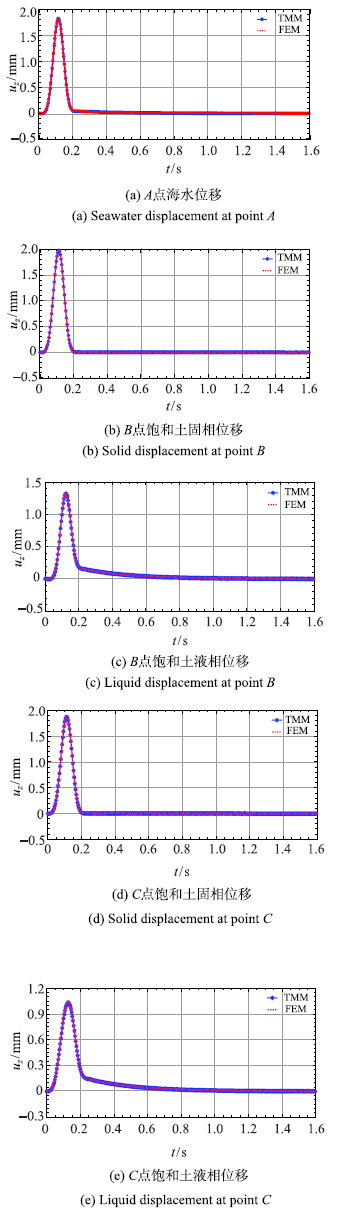Fig.9   Displacement of seawater-saturated soil system for P wave incidence

### 图10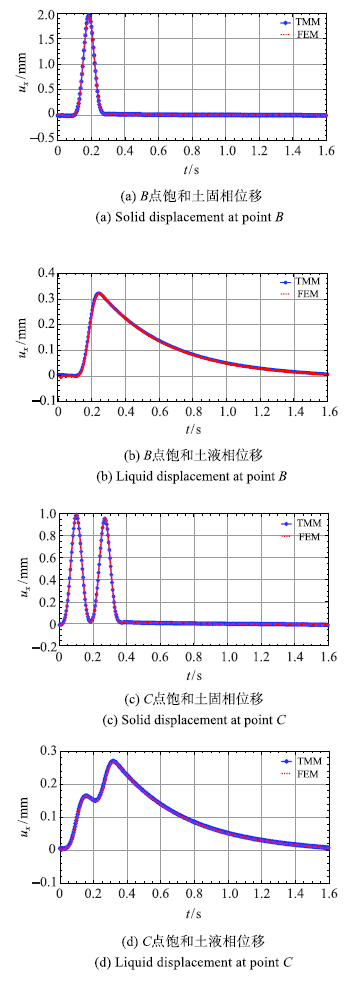Fig.10   Displacement of seawater-saturated soil system for SV wave incidence

### 图11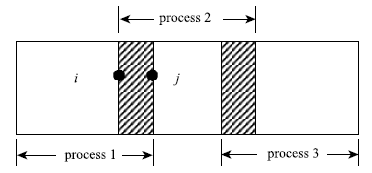Fig.11   Schematic diagram of parallel computation

### 图12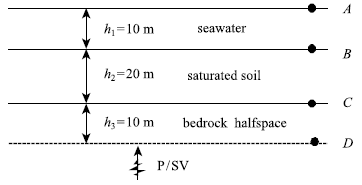Fig.12   Seawater-saturated soil-bedrock

### 图13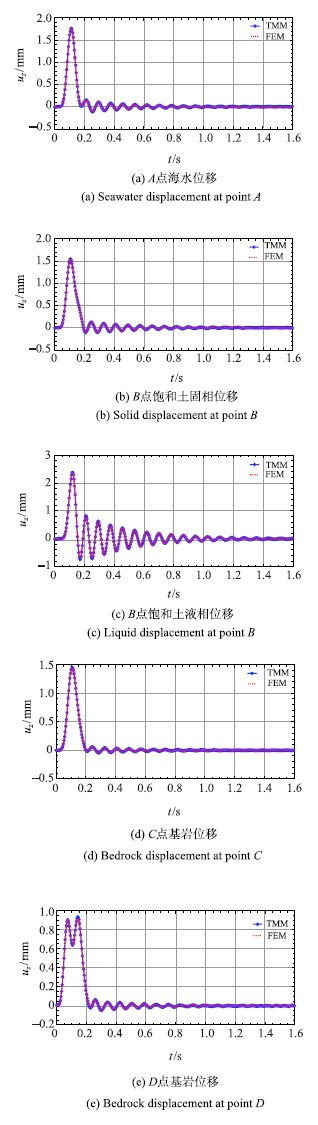Fig.13   Displacement of seawater-saturated soil-bedrock system for P wave incidence

### 图14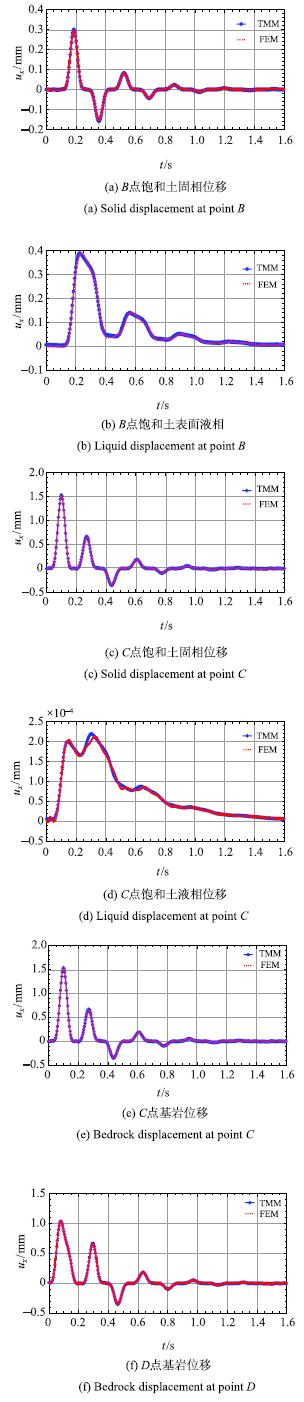Fig.14   Displacement of seawater-saturated soil-bedrock system for SV wave incidence

## 3 结 论

The authors have declared that no competing interests exist.

## 参考文献 原文顺序 文献年度倒序 文中引用次数倒序 被引期刊影响因子

Nakamura T, Takenaka H, Okamoto T , et al.

FDM Simulation of seismic-wave propagation for an aftershock of the 2009 Suruga Bay earthquake: Effects of ocean-bottom topography and seawater layer

Bulletin of Seismological Society of America, 2012,102(6):2420-2435

URL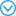ABSTRACT Feasibility studies on simulating seismic-wave propagation in media for a suboceanic earthquake, including both land and ocean-bottom topographies and a seawater layer, are scarce. Some of the conventional staggered-grid finite-difference method (FDM) simulations use a simplified structure model without a seawater layer and with a flat ocean-bottom topography. In this study, we apply our heterogeneity, oceanic layer, and topography scheme (HOT)-FDM and a 3D structure model including land and ocean-bottom topographies, a seawater layer, and a fluid-solid boundary condition to an aftershock (M-w 5.8) of the 2009 Suruga Bay earthquake. We attempt to reproduce observations at seismic stations near the coast and then simulate waveforms in the ocean-bottom stations. Our results show that a large difference between the cases with and without topographies can be seen in the coda part after the S wave in the simulated waveforms in terms of amplitudes and elongations. The synthetic waveforms in the model with topographies are in agreement with the observed waveforms. Our results also show that a significant difference in the amplification of the coda part between the cases with and without the seawater layer can be found at the ocean-bottom stations. This coda part is the S-and Rayleigh-wave propagation associated with the ocean and the underlying sediment layers. Our results show that a realistic model with topographies and a seawater layer is needed in FDM simulations in order to precisely reproduce observed waveforms or predict seismic motion for a suboceanic earthquake.

Petukhin A, Iwata T, Kagawa T .

Study on the effect of the oceanic water layer on strong ground motion simulations

Earth Planets Space, 2010,62:621-630

URLWe studied the effect of the oceanic water layer on strong ground motion simulations. Source faults of subduction zone earthquakes, such as the Nankai-Tonankai earthquake, West Japan, are situated in the offshore area, under a thick water layer. The necessity of including the oceanic water layer in the velocity model for simulations employing the finite difference method is debated by many researchers, and consideration given to the possibility of neglecting by this layer, which would reduce the computation time and stabilize the calculations. Although the oceanic water layer has a low velocity and density, it can affect surface wave generation. In this study, for demonstration purposes, we calculated and compared strong ground motions from three source fault models, placed into the boundary between the crust and subducting plate, where source rupture of the Tonankai earthquake is expected. Simulations were made for two realistic three-dimensional velocity models: without and with the oceanic water layer. The model without the oceanic water layer was constructed simply by subtracting the depth of the oceanic layer from the depth of all velocity interfaces under the ocean. This procedure keeps the thickness of the layers (oceanic sediments, surface low-velocity layer, upper crust, and lower crust) the same as in the model with the oceanic layer and reduces simulation errors. Simulations were made for the set of sites on a line across the subduction zone and directed to the Osaka basin. The results show that the water layer has a strong effect on the fundamental mode of the Rayleigh wave, which can be generated by the shallow (approx. 5 km) source. Considering that all asperities of the expected Nankai-Tonankai earthquake are deep (> 10 km), we conclude that the effect of the water layer can be neglected for ground motions at land sites.

Jianhong Y ,

Seismic response of poroelastic seabed and composite breakwater under strong earthquake loading

Bulletin of Earthquake Engineering, 2012,10:1609-1633

URLAbstract 61 ” as the governing equation for porous seabed foundation, the seismic response of a composite breakwater and its porous seabed foundation under the seismic wave recorded in the Japan 311 off the pacific coast of Tohoku earthquake (M L =029.0) is investigated using a FEM numerical model. The numerical results indicate that the seismic response of composite breakwater is very strong in the earthquake process. The amplification of the input seismic wave occurs both in seabed foundation and composite breakwater; and this amplification is positively related to the buried depth of points. The horizontal seismic response is much strong than the vertical seismic response. The seismic wave induced excess pore pressure and effective stresses in seabed foundation vibrates; the vibration amplitude is also positively related to the buried depth of points. Under strong seismic loading, the surface region of seabed foundation could liquefy. The parametric study shows that the young’s modulus of seabed foundation has significant effect on the seismic response of composite breakwater.

Cheng XS, Xu WW, Yue CQ , et al.

Seismic response of fluid-structure interaction of undersea tunnel during bidirction earthquake

Ocean Engineering, 2014,75:64-70

Jin HL, Seo SI, Mun HS .

Seismic behaviors of a floating submerged tunnel with a rectangular cross-section

Ocean Engineering, 2016,127:32-47

URL61Two-dimensional seismic behaviors of a submerged floating tunnel (SFT) are examined.61The effects of seawater upon the seismic behaviors of an SFT system are investigated.61The depth of the ocean water is one of the important factors for the SFT system.61Behaviors of the SFT system are affected by the location of the tunnel in the water.61The energy absorption by seabed influences the dynamic responses of the SFT system.

Farhat C, Lesoinne M ,

LeTallec P. Load and motion transfer algorithms for fluid/structure interaction problems with non-matching discrete interfaces: Momentum and energy conservation, optimal discretization and application to aeroelasticity

Computer Methods in Applied Mechanics & Engineering, 1998,157(1):95-114

Farhat C, Lesoinne M .

Two effcient staggered algorithms for serial and parallel solution of three-dimensional nonlinear transient aeroelastic problems

Computer Methods in Applied Mechanics & Engineering, 2000,182:499-515

URLPartitioned procedures and staggered algorithms are often adopted for the solution of coupled fluid/structure interaction problems in the time domain. In this paper, we overview two sequential and parallel partitioned procedures that are popular in computational nonlinear aeroelasticity, and address their limitation in terms of accuracy and numerical stability. We propose two alternative serial and parallel staggered algorithms for the solution of coupled transient aeroelastic problems, and demonstrate their superior accuracy and computational efficiency with the flutter analysis of the AGARD Wing 445.6. We contrast our results with those computed by other investigators and validate them with experimental data.

Farhat C, Zee KGVD, Geuzaine P .

Provably second-order time-accurate loosely-coupled solution algorithms for transient nonlinear computational aeroelasticity

Computer Methods in Applied Mechanics & Engineering, 2006,195(17):1973-2001

URLA methodology for designing formally second-order time-accurate and yet loosely-coupled partitioned procedures for the solution of nonlinear fluid tructure interaction (FSI) problems on moving grids is presented. Its key components are a fluid time-integrator that is provably second-order time-accurate on moving grids, the midpoint rule for advancing in time the solution of the structural dynamics equations of motion, a second-order structure predictor for bypassing the inner-iterations encountered in strongly-coupled solution procedures, and a carefully designed algorithm for time-integrating the motion of the fluid-mesh. Following this methodology, two different loosely-coupled schemes are constructed for the solution of transient nonlinear FSI problems and proved to be second-order time-accurate. Three-dimensional numerical results pertaining to the simulation of the aeroelastic response to a gravity excitation of a complete F-16 configuration are also presented. In addition to confirming the theoretical results discussed in this paper, these numerical results highlight a very stable behavior of the designed loosely-coupled partitioned procedures.

Bathe KJ, Zhang H .

Finite element developments for general fluid flows with structural interactions

International Journal for Numerical Methods in Engineering, 2010,60(1):213-232

URLThe objective in this paper is to present some developments for the analysis of Navier-Stokes incompressible and compressible fluid flows with structural interactions. The incompressible fluid is discretized with a new solution approach, a flow-condition-based interpolation finite element scheme. The high-speed compressible fluids are solved using standard finite volume methods. The fluids are fully coupled to general structures that can undergo highly non-linear response due to large deformations, inelasticity, contact and temperature. Particular focus is given on the scheme used to couple the fluid media with the structures. The fluids can also be modelled as low-speed compressible or slightly compressible media, which are important models in engineering practice. Some solutions obtained using ADINA are presented to indicate the analyses that can be performed

Degroote J, Haelterman R, Annerel S , et al.

Performance of partitioned procedures in fluid-structure interaction

Computers & Structures, 2010,88(7):446-457

URLPartitioned simulations of fluid–structure interaction can be solved for the interface’s position with Newton–Raphson iterations but obtaining the exact Jacobian is impossible if the solvers are “black boxes”. It is demonstrated that only an approximate Jacobian is needed, as long as it describes the reaction to certain components of the error on the interface’s position. Based on this insight, a quasi-Newton coupling algorithm with an approximation for the inverse of the Jacobian (IQN-ILS) has been developed and compared with a monolithic solver in previous work. Here, IQN-ILS is compared with other partitioned schemes such as IBQN-LS, Aitken relaxation and Interface-GMRES(R).

Hou G, Wang J, Layton A .

Numerical methods for fluid-structure Interaction-A review

Communications in Computational Physics, 2012,12(2):337-377

URLThe interactions between incompressible fluid flows and immersed structures are nonlinear multi-physics phenomena that have applications to a wide range of scientific and engineering disciplines. In this article, we review representative numerical methods based on conforming and non-conforming meshes that are currently available for computing fluid-structure interaction problems, with an emphasis on some of the recent developments in the field. A goal is to categorize the selected methods and assess their accuracy and efficiency. We discuss challenges faced by researchers in this field, and we emphasize the importance of interdisciplinary effort for advancing the study in fluid-structure interactions.

Habchi C, Russeil S, Bougeard D , et al.

Partitioned solver for strongly coupled fluid-structure interaction

Computers & Fluids, 2013,71(1):306-319In this work a fluid-structure interaction solver is developed in a partitioned approach using block Gauss-Seidel implicit scheme. Finite volume method is used to discretize the fluid flow problem on a moving mesh in an arbitrary Lagrangian-Eulerian formulation and by using an adaptive time step. The pressure-velocity coupling is performed by using the PIMPLE algorithm, a combination of both SIMPLE and PISO algorithms, which permits the use of larger time steps in a moving mesh. The structural elastic deformation is analyzed in a Lagrangian formulation using the St. Venant-Kirchhoff constitutive law, for non-linear large deformations. The solid structure is discretized by the finite volume method in an iterative segregated approach. The automatic mesh motion solver is based on Laplace smoothing equation with variable mesh diffusion. The strong coupling between the different solvers and the equilibrium on the fluid-structure interface are achieved by using an iterative implicit fixed-point algorithm with dynamic Aitken's relaxation method. The solver, which is called vorflexFoam, is developed using the open source C++ library OpenFOAM. The solver is validated on two different benchmarks largely used in the open literature. In the first one the structural deformation is induced by incompressibility. The second benchmark consists on a vortex excited elastic flap in a Von Karman vortex street. Finally, a more complex case is studied including two elastic flaps immersed in a pulsatile flow. The present solver detects accurately the interaction between the complex flow structures generated by the flaps and the effect of the flaps oscillations between each other. (c) 2012 Elsevier Ltd. All rights reserved.

Mehl M, Uekermann B, Bijl H , et al.

Parallel coupling numerics for partitioned fluid-structure interaction simulations

Computers & Mathematics with Applications, 2016,71(4):869-891

URLWithin the last decade, very sophisticated numerical methods for the iterative and partitioned solution of fluid tructure interaction problems have been developed that allow for high accuracy and very complex scenarios. The combination of these two aspects ccuracy and complexity emands very high computational grid resolutions and, thus, high performance computing methods designed for massively parallel hardware architectures. For those architectures, currently used coupling methods, which mainly work with a staggered execution of the fluid and the structure solver, i.e., the execution of one solver after the other in every outer iteration, lead to severe load imbalances: if the flow solver, e.g., scales on a very large number of processors but the structural solver does not due to its limited amount of data and required operations, almost all processors assigned to the coupled simulations are idle during the execution of the structure solver. We propose two new iterative coupling methods that allow for the simultaneous execution of flow and structure solvers. In both cases, we show that pure fixed-point iterations based on the parallel execution of the solvers do not lead to good results, but the combination of parallel solver execution and so-called quasi-Newton methods yields very efficient and robust methods. Those methods are known to be very efficient also for the stabilization of critical scenarios solved with the standard staggered solver execution. We demonstrate the competitive convergence of our methods for various established benchmark scenarios. Both methods are perfectly suited for use with black-box solvers because the quasi-Newton approach uses solely input and output information of the solvers to approximate the effect of the unknown Jacobians that would be required in a standard Newton solver.

Bungartz HJ, Lindner F, Gatzhammer B , et al.

preCICE-A fully parallel library for multi-physics surface coupling

Computers & Fluids, 2016,141:250-258

URLIn the emerging field of multi-physics simulations, we often face the challenge to establish new connections between physical fields, to add additional aspects to existing models, or to exchange a solver for one of the involved physical fields. If in such cases a fast prototyping of a coupled simulation environment is required, a partitioned setup using existing codes for each physical field is the optimal choice. As accurate models require also accurate numerics, multi-physics simulations typically use very high grid resolutions and, accordingly, are run on massively parallel computers. Here, we face the challenge to combine flexibility with parallel scalability and hardware efficiency. In this paper, we present the coupling tool preCICE which offers the complete coupling functionality required for a fast development of a multi-physics environment using existing, possibly black-box solvers. We hereby restrict ourselves to bidirectional surface coupling which is too expensive to be done via file communication, but in contrast to volume coupling still a candidate for distributed memory parallelism between the involved solvers. The paper gives an overview of the numerical functionalities implemented in preCICE as well as the user interfaces, i.e., the application programming interface and configuration options. Our numerical examples and the list of different open-source and commercial codes that have already been used with preCICE in coupled simulations show the high flexibility, the correctness, and the high performance and parallel scalability of coupled simulations with preCICE as the coupling unit.

Banks JW, Henshaw WD, Kapila AK , et al.

An added-mass partition algorithm for fluid-structure interactions of compressible fluids and nonlinear solids

Journal of Computational Physics, 2016,305(C):1037-1064

URLWe describe an added-mass partitioned (AMP) algorithm for solving fluid–structure interaction (FSI) problems involving inviscid compressible fluids interacting with nonlinear solids that undergo large rotations and displacements. The computational approach is a mixed Eulerian–Lagrangian scheme that makes use of deforming composite grids (DCG) to treat large changes in the geometry in an accurate, flexible, and robust manner. The current work extends the AMP algorithm developed in Banks et al.  for linearly elasticity to the case of nonlinear solids. To ensure stability for the case oflightsolids, the new AMP algorithm embeds an approximate solution of a nonlinear fluid–solid Riemann (FSR) problem into the interface treatment. The solution to the FSR problem is derived and shown to be of a similar form to that derived for linear solids: the state on the interface being fundamentally an impedance-weighted average of the fluid and solid states. Numerical simulations demonstrate that the AMP algorithm is stable even for light solids when added-mass effects are large. The accuracy and stability of the AMP scheme is verified by comparison to an exact solution using the method of analytical solutions and to a semi-analytical solution that is obtained for a rotating solid disk immersed in a fluid. The scheme is applied to the simulation of a planar shock impacting a light elliptical-shaped solid, and comparisons are made between solutions of the FSI problem for a neo-Hookean solid, a linearly elastic solid, and a rigid solid. The ability of the approach to handle large deformations is demonstrated for a problem of a high-speed flow past a light, thin, and flexible solid beam.

Basting S, Quaini A, Glowinski R .

Extended ALE Method for fluid-structure interaction problems with large structural displacements

Journal of Computational Physics, 2016,331(C):312-336

URLStandard Arbitrary Lagrangian-Eulerian (ALE) methods for the simulation of fluid-structure interaction (FSI) problems fail due to excessive mesh deformations when the structural displacement is large. We propose a method that successfully deals with this problem, keeping the same mesh connectivity while enforcing mesh alignment with the structure. The proposed Extended ALE Method relies on a variational mesh optimization technique, where mesh alignment with the structure is achieved via a constraint. This gives rise to a constrained optimization problem for mesh optimization, which is solved whenever the mesh quality deteriorates. The performance of the proposed Extended ALE Method is demonstrated on a series of numerical examples involving 2D FSI problems with large displacements. Two way coupling between the fluid and structure is considered in all the examples. The FSI problems are solved using either a Dirichlet-Neumann algorithm, or a Robin-Neumann algorithm. The Dirichlet-Neumann algorithm is enhanced by an adaptive relaxation procedure based on Aitken's acceleration. We show that the proposed method has excellent performance in problems with large displacements, and that it agrees well with a standard ALE method in problems with mild displacement.

Biot MA .

Theory of propagation of elastic waves in a fluid-saturated porous solid

Acoust Soc Am, 1956,28:168-191

Biot MA .

Mechanics of deformation and acoustic propagation in porous media

Journal of Applied Physics, 1962,33(4):1482-1498

URLA unified treatment of the mechanics of deformation and acoustic propagation in porous media is presented, and some new results and generalizations are derived. The writer's earlier theory of deformation of porous media derived from general principles of nonequilibrium thermodynamics is applied. The fluid﹕olid medium is treated as a complex physicalヽhemical system with resultant relaxation and viscoelastic properties of a very general nature. Specific relaxation models are discussed, and the general applicability of a correspondence principle is further emphasized. The theory of acoustic propagation is extended to include anisotropic media, solid dissipation, and other relaxation effects. Some typical examples of sources of dissipation other than fluid viscosity are considered.

Komatitsch D, Barnes C, Tromp J .

Wave propagation near a fluid-solid interface: A spectral-element approach

Geophysics, 2000,65(2):623-631

Link G, Kaltenbacher M, Breuer M , et al.

A 2D finite-element scheme for fluid-solid-acoustic interactions and its application to human phonation

Computer Methods in Applied Mechanics & Engineering, 2009,198(41):3321-3334

URLWe present a recently developed approach for the modeling of fluid–solid–acoustic interaction problems. For the efficient numerical solution of the coupled three-field problem we apply the finite-element method. The mechanical and the acoustic fields are approximated by a standard Galerkin scheme. A residual-based stabilization method is chosen for the fluid field. The interaction of the Eulerian fluid field with the Lagrangian mechanical field is based on the Arbitrary-Lagrangian–Eulerian (ALE) method and is iteratively coupled in a strong sense. The solid–acoustic interaction is based on continuum mechanics, and the fluid–acoustic coupling on Lighthill’s analogy. The new steps of our scheme are verified through validation examples. Finally, a fluid–solid–acoustic simulation of the human phonation process is presented based on a realistic model.

URL( Li Weihua .

Seismic Response analysis of immersed tube tunnels considering water saturated soil site structure coupling

Journal of Disaster Prevention and Mitigation Engineering, 2010,30(6):607-613(in Chinese))

URL( Liao Zhenpeng. Introduction to Wave Motion Theories in Engineering(2nd edn). Beijing: Science Press, 2002: 136-285(in Chinese))

URL( Xing Haojie, Li Hongjing .

Implementation of multi-transmitting boundary condition for wave motion simulation by spectral element method: Two dimension case

Chinese Journal of Theoretical and Applied Mechanics, 2017,49(4):894-906 (in Chinese))

URLURL( Gu Lin, Liu Jingbo, Du Xiuli .

Three-dimensional uniform viscoelastic artificial boundary and equivalent viscoelastic boundary element

Journal of Engineering Mechanics, 2007,24(12):31-37(in Cinese))

URLURL( Liu Jingbo ,

BaoXin, Tan Hui, et al. Dynamical artificial boundary for fluid medium in wave motion problems

Chinese Journal of Theoretical and Applied Mechanics, 2017,49(6):1418-1427 (in Chinese))

URLMagsci( Zhao Yuxin, Chen Shaolin .

Discussion on the matrix propagator method to analyze the response od saturated layered media

Chinese Journal of Theoretical and Applied Mechanics, 2016,48(5):1145-1158 (in Chinese))

MagsciURL( Liu Jingbo, Tan Hui, Bao Xin , et al.

The seismic wave input method for soil-structure dynamic interaction analysis based on the substructure of artificial boundaries

Chinese Journal of Theoretical and Applied Mechanics, 2018,50(1):32-43 (in Chinese))

URLMagsci( Chen Shaolin, Liao Zhenpeng, Chen Jin .

Decoupling method for near-field wave simulation of two-phase media

Journal of Geophysics, 2005,48(4):909-917 (in Chinese))

MagsciDeresiewicz H, Rice JT .

The effect of boundaries on wave propagation in a liquid-filled porous solid: V. Transmission across a plane interface

Bull Seis Soc Am, 1964,54(1):409-416

Deresiewicz H .

The effect of boundaries on wave propagation in a liquid-filled porous solid: VII. Surface waves in a half-space in the presence of a liquid layer

Bull Seis Soc Am, 1964,54(1):425-430

Thomson WT .

Transmission of elastic waves through a stratified solid media

Journal of Applied Physics, 1950,21:89-93

URLThe transmission of a plane elastic wave at oblique incidence through a stratified solid medium consisting of any number of parallel plates of different material and thickness is studied theoretically. The matrix method is used to systematize the analysis and to present the equations in a form suitable for computation.

The dispersion of surface waves on multilayered media

Bull Seismol Soc Am, 1953,43:17-34

URLA matrix formalism developed by W. T. Thomson is used to obtain the phase velocity dispersion equations for elastic surface waves of Rayleigh and Love type on multi-layered solid media. The method is used to compute phase and group velocities of Rayleigh waves for two assumed three-layer and one two-layer model of the earth's crust in the continents. The computed group velocity curves are compared with published values of the group velocities at various frequencies of Rayleigh waves over continental paths. The scatter of the observed values is larger than the difference between the three computed curves. It is believed that not all of this scatter is due to observational errors but probably represents a real horizontal heterogeneity of the continental crusts. (Author)

P-SV波入射时海水-层状海床体系的自由场分析

. 振动工程学报, 2018, 录用

( Ke Xiaofei, Chen Shaolin, Zhang Hongxiang .

Freefield analysis of seawater-layered seabed system at P-SV wave incident

Journal of Vibration Engineering, 2018, Accepted (in Chinese))

/

 〈〉